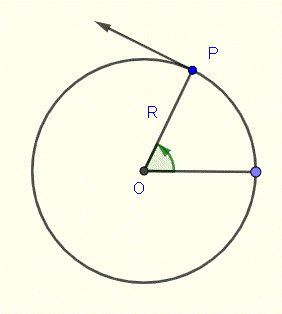# Angular, Linear Speeds and Revolutions Calculator

An online angular and linear speeds, and revolutions calculator in a system that is moving along a circular path and at a constant speed. This calculator converts the number of revolutions per minutes (RPM) of a point P rotating at a distance R from the center of rotation O, into radians per second and meters per second. It also converts angular and linear speed into revolutions per minute.
If rpm is the number of revolutions per minute, then the angular speed ω in radians per second and the linear speed v are given by
ω = rpm � 2 π / 60 (radians per second)
v = ω � R (meters/second)## 1 - Use of Angular, Linear Speeds and Revolutions Calculator Given RPM

Enter the RPM ( in revoluitons per minute) and the radius R (in meters) as positive real numbers then press "Calculate Angular and Linear Speeds".

 RPM = 1000 revolutions per minute Radius: R = 0.1 meters Decimal Places = 2 Angular Speed: ω = radians / second Linear Speed: v = meters / second

## 2 - Use of Angular, Linear Speeds and revolutions Calculator Given Angular Speed

Enter the angular speed ω and the radius R (in meters) as positive real numbers and press "Calculate Linear Speed and RPM".

 Angular Speed: ω = = 1000 radians per second Radius R = 0.1 meters Decimal Places = 2 Linear Speed: v = meters / second RPM = rotations / minute

## 3 - Use of Angular, Linear Speeds and Revolutions Calculator Given Linear Speed

Enter the linear speed v and the radius R (in meters) as positive real numbers and press "Calculate Angular Speed and RPM".

 Linear Speed: v = = 1000 meters per second Radius R = 0.1 meters Decimal Places = 2 Angular Speed: ω = radians / second RPM = revolutions / minute